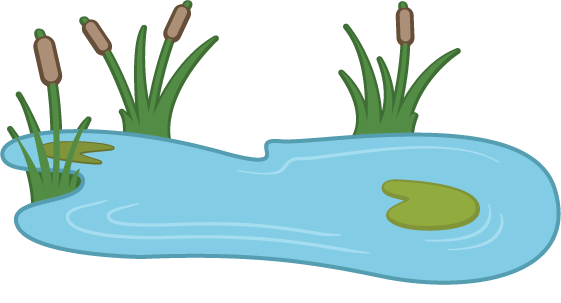Problems
231 - Pond Digging (II)

Pond Digging (II)

Time Limit: 1 sec

The Problem

You all may be remembered Mrs. Rahman who wanted to dig a pond in her rectangular plot. After talking Ali suddenly a thought came to her mind what will be the area of the edge.  She made a way to calculate this. Can you find the way which she used to calculate the area of edge?The Input

The input file contains four integer numbers length, L (L<=500) and width, W (W<=400) of the plot and length, l (l<=5) and width, w (W<=4) of the border.

The Output

The output will show the area of the edge in a separate line.

Sample Input

100 80 3 2

Sample Output

832

Problem Setter: Samia Safa Ahmed

Special thanks to Shahin ShamS